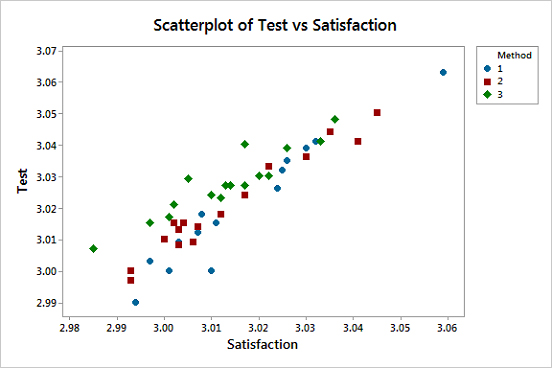# Manova Test

Multivariate analysis of variance (MANOVA) can be termed as a univariate analysis of variance (ANOVA). In ANOVA, we test the statistical differences on one continuous dependant variable by an independent grouping variable. Analysis of Variance statistically tests the variations between 3 or additional clusters suggests that however with only 1 dependant variable at a time. In the MANOVA test, there is an extension of analysis as here we take into account multiple continuous dependant variables and bundle them into a composite variable. In testing the statistical significance of the mean MANOVA uses the covariance between outcome variables. Hence, it is done when there are two or more dependant variables. There are many such benefits of MANOVA over ANOVA.

## How MANOVA Assesses the Data?

Below is the scatter plot graph in between the test and satisfaction scores. Here we will note what patterns we can find between the dependant variables and how they are related to the teaching method. This graph depicts a positive correlation between test scores and Satisfaction.

As the satisfaction of students increases, the test scores tend to increase. MANOVAcan test this pattern statistically to ensure that it’s not present by chance. The MANOVA model fits in your software so that Method is the independent variable and Satisfaction and Test are the dependant variables.

MANOVA represents statistically significant results while by one-way ANOVA results and graphs there is nothing of interest.As the satisfaction of students increases, the test scores tend to increase. MANOVA can test this pattern statistically to ensure that it’s not present by chance. , MANOVA model fits in your software so that Method is the independent variable and Satisfaction and Test are the dependent variables.

MANOVA represents statistically significant results while by one-way ANOVA results and graphs there is nothing of interest.

## Assumptions :-

1. As per MANOVA, there is just a random selection of samples and there is no particular pattern.
2. As per MANOVA, the independent variables are categorical and the dependent variables are continuous.
3. There is an absence of multicollinearity, as per Tabachnick & Fidell (2012), no correlation should be above r = .90.
4. Variance is homogeneous.

## Key concepts and terms :-

1. Levene’s Test of Equality of Variance: This test is performed to check if the variance between independent variable groups are equal or not.
2. sBox’s M Test: This test is performed to know the equality of covariance between the groups. This test is highly sensitive and is determined at α = .001.
3. Partial eta square: This test shows the variance explained by the independent variable.
4. Post hoc test: The differences between the groups are identified by the Post hoc test.